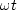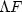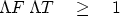Next: A misinterpretation of the Up: Resolution and random signals Previous: Resolution and random signals

# TIME-FREQUENCY RESOLUTION

A consequence of Fourier transforms being built fromis that scaling a function to be narrower in one domain scales it to be wider in the other domain. Scalingimplies inverse scaling of t to keep the productconstant. For example, the FT of a rectangle is a sinc. Making the rectangle narrower broadens the sinc in proportion becauseis constant. A pure sinusoidal wave has a clearly defined frequency, but it is spread over the infinitely long time axis. At the other extreme is an impulse function (often called a delta function), which is nicely compressed to a point on the time axis but contains a mixture of all frequencies. In this section we examine how the width of a function in one domain relates to that in the other.

By the end of the section, we will formalize this into an inequality:

For any signal, the time durationand the spectral bandwidthare related by(1)
This inequality is the uncertainty principle.

Since we are unable to find a precise and convenient analysis for the definitions ofand,the inequality (1) is not strictly true. What is important is that rough equality in (1) is observed for many simple functions, but for others the inequality can be extremely slack (far from equal). Strong inequality arises from all-pass filters.

An all-pass filter leaves the spectrum unchanged, and henceunchanged, but it can spread out the signal arbitrarily, increasingarbitrarily. Thus the time-bandwidth maximum is unbounded for all-pass filters. Some people say that the Gaussian function has the minimum product in  (1), but that really depends on a particular method of measuringand.Next: A misinterpretation of the Up: Resolution and random signals Previous: Resolution and random signals
Stanford Exploration Project
10/21/1998